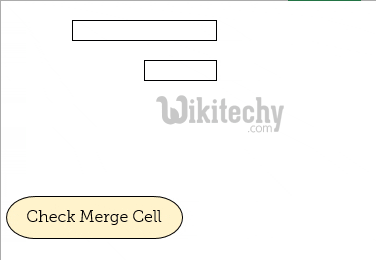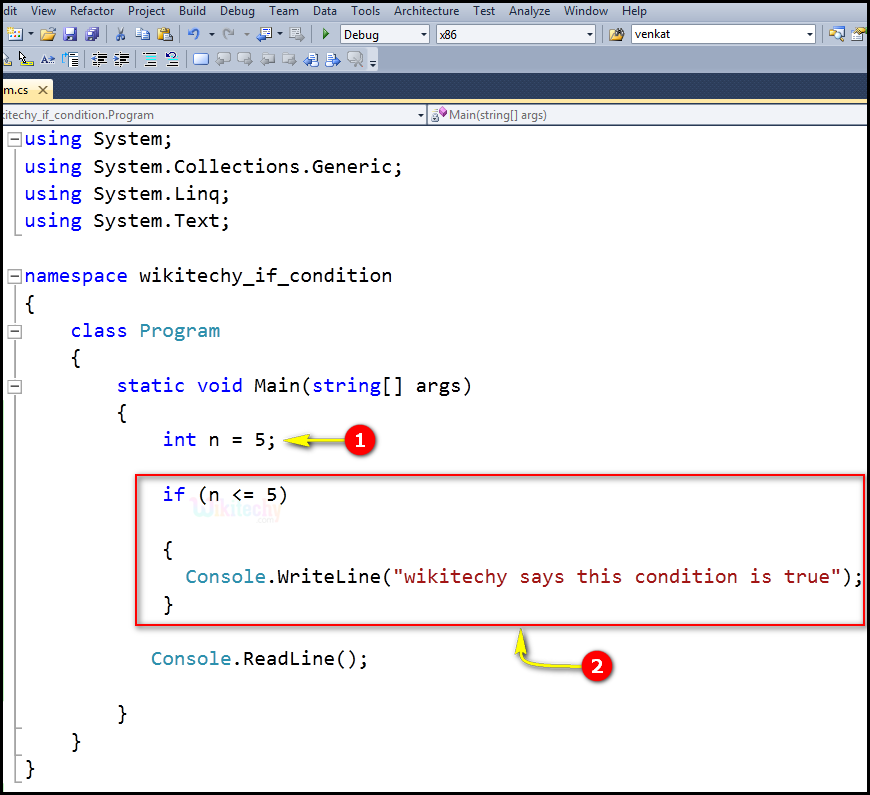# C# if statement | c# if | if c# - c# - c# tutorial - c# netC# If Statement

## What is if statement in C# ?

• In C#, an if statement consists of a Boolean expression followed by one or more statements.## Syntax:if statement in c#

## Code Explanation:1. Here n = 5 is an integer variable value as 5.
2. Here, if (n <= 5) expression specifies the n =5 is less than, or equal to 5 i.e., (5 <= 5), which executes the statement "wikitechy says this condition is true".

## Sample C# examples - Output :1. Here the statement executes the if condition by printing the statement "Wikitechy says this condition is true".